# The Ascendant, the Sun and the Moon Acting as a PID Controller in the Astrological Chart

The three most important factors in any astrological chart are the Ascendant, the Sun and the Moon. The way they operate can be explained with an analogy from the engineering field. The idea came to me while I was presenting a lecture to a group of professional engineers and technicians on PID loop tuning. The principle of any loop tuning is to keep the process variable (PV) as close as possible to some preset value, called set point (SP), in spite of constantly changing disturbances. If we regard the whole setup as a black box, then its output (which is analogous to the process variable), has to remain at all times as close as possible to the set point, regardless of constantly changing input conditions. Let’s take the operation of a typical air-conditioning system as an example. The temperature of the air that blows out from the air-conditioner has to be such value, that as an end result, the room temperature is always kept constant and close to the set point, regardless of the changing ambient temperature.

The human behaviour can be described to a large extent on the basis of a such black box operation. In the ideal case scenario, the people’s behaviour should be steady, and closely approximating their set point, which in this case is equivalent to their preordained spiritual path. The tapestry of life situations in which people find themselves are analogous to the constantly changing input signal. The only difference is in the complexity of the function that has to be calculated in the black box in order to produce the desired output. When we are dealing with an air-conditioning system, we have to take into account one variable only and the mathematical formula, used for control of the temperature is relatively simple. When we are dealing with human beings, we have to take into account thousands of variables and the formula becomes extremely complex. And yet, we still can draw parallels between the two black boxes. We still can roughly delineate the main life progressions of a person, as a function of his spiritual development.

In order to do that, we need to concentrate initially only on the three most important factors in any astrological chart – these are the Ascendant, the Sun and the Moon. Later on, as we become more experienced, we can add more features. In this way we can gain a better resolution of the picture that we are getting. However, as a starter the three factors mentioned are quite adequate. If we proceed in this manner, we can make an analogy between the operations of any pre-programmed machine and a human being. We can discover some striking similarities. We can see that similarly to a pre-programmed machine, a human is also pre-programmed by birth to follow a specific life program. The only difference is that humans possess a small percentage of free will as well. This fraction can be anywhere between 5 – 20% depending on the person’s spiritual level of development. This unknown parameter can modify the final output of their behaviour to a certain extent for good or for bad. The explanation, given below can be better understood by people who have at least some engineering or technical background. Some familiarity of the PID loops would be ideal.

Before we can make the above-mentioned analogy, let us remind ourselves the basics of the PID control. PID stands for proportional-integral-derivative, which are the three components comprising any automated controller. This type of regulation lays the foundations of almost any automated process in industry, production, households and so forth. The block diagram of closed loop PID controller is given below.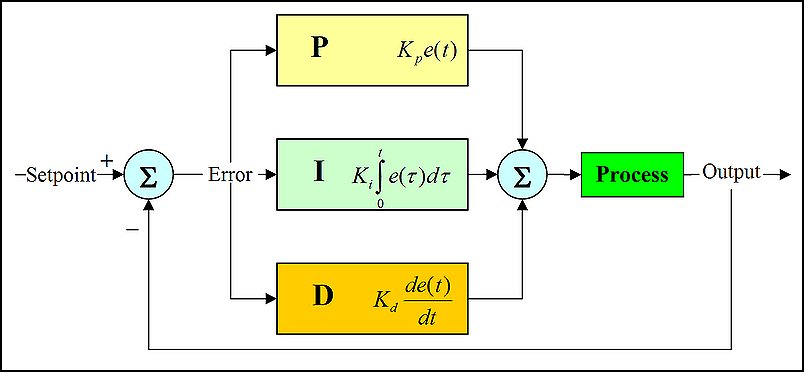The idea is to measure the output of the process and to enter this signal back to the input of the controller. There this signal is compared to the set point. The difference between the two signals is called an error. Thus:

ERR = SP – PV

where ERR is the error, SP is the set point and PV is the process variable.

Let’s go back to the example of the air-conditioning system that we used before. Here, the SP is the desired room temperature (let’s say 20°C), the PV at the moment is say 15°C, which is lower from what it is expected. Therefore, the error is 5°C. The feedback is provided usually by a thermistor. This device changes its resistance according to the ambient temperature, thus feeding back a signal to the input of the controller.

It is logical to think that a larger error will produce a larger output of the controller. In this way, the error would be eliminated faster. This type of control is called proportional. Here the output of the controller is calculated as the product of the error and some coefficient, which we call proportional gain. The formula for the proportional gain is given below.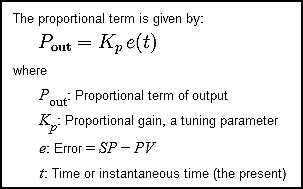This is the main type of correction. The output of the controller is directly proportional to the amount of error and it is directly proportional to the gain, hence the term proportional controller. Lager error produces larger output. Larger gain also produces larger output. One could think that large gain would solve all our problems, as large gain would make the controller react fast and vigorous. Unfortunately, in reality, the situation is never that simple. Very high gain of the proportional controller also means instability and over-control. The controller may start changing its output constantly between its two highest extremes and thus can become very unstable. This phenomenon is known as “hunting”.

But even if we use a nominal value for the gain, the proportional controller alone can never eliminate the error completely. It ceases to work as soon as the process variable draws near the SP. Then, it establishes a fixed output. The existing error (albeit small) is then omnipresent in the system and can never be reduced to zero. This small error is called an offset. The biggest problem of the proportional controller lies in the fact that it is unable to eliminate the offset completely. Similarly, when people are trying consciously to eliminate the error between what it is set for them as a spiritual set point from above and the real state of affairs they are experiencing on the earth plane at the moment, they can never eliminate this error completely. The action of the proportional controller is similar to the action of the Sun in the astrological chart of a person.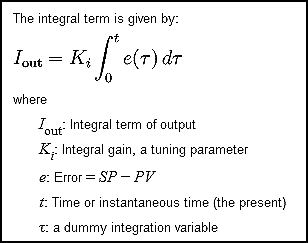The inability of the proportional controller to eliminate the error completely can be overcome if an integral controller is introduced. Its output is calculated as the product of the integral of the error over some time and some coefficient. In this manner, the longer the error is present on the input of the system, the stronger the signal becomes at the output. This means that even minimal errors will produce significant output, if they are present on the input for long enough. The main advantage of the integral controller lies in is its ability to eliminate the offset completely. This type of device looks at the past. It builds up and accumulates errors. The greater the level of past mistakes, the stronger reaction it produces. In a similar fashion, we can say that our instinctive reactions in situations are an integral product of all our mistakes done in the past, including all our past lives. Therefore the action of the integral controller is directly related to the action of the Moon in the astrological chart of a person.

However, the integral of a sine is minus cosine. This means that if we input a sinusoidal signal to the integral controller, we will get an output signal that is lagging the input signal on 90°. As the output is fed back to the input, the lagging output will be added to the input sinusoidal wave, thus producing a highly distorted output signal. As a result, the system can become much more unstable to what it used to be before. To overcome this problem we can introduce a differential controller. Its output is calculated as a derivative of the error, multiplied by some coefficient. The derivative of sine is cosine, thus the output signal this time will lead the input on 90°. In such way the derivative action can counteract the integral action. Thus, the instability introduced by the integral controller can be eliminated.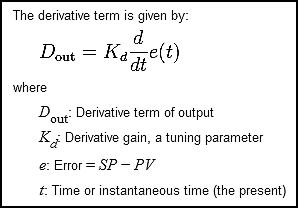From a physical point of view, the derivative controller reacts only on the rate of change of error. Even if a huge error is present between the SP and the PV, the derivative controller is not going to take any action, if it does not “see” any change in their values. On the other hand, if the absolute value of the error is minimal, but the rate of change is high, the derivative controller will produce a significant output signal. In other words, the derivative controller faces the future. It acts on the rate of change of error only. It sees a certain tendency and tries to eliminate future problems, which this tendency can bring, if it is left unattended. In an astrological chart of a person, the Ascendant is that point that determines what we would like to become and how we would like to be seen by the other people in the future. How we look today is determined by our Sun sign. However, if we want to determine what is our driving force which gears us towards the future, then we have to look at the Ascendant. Therefore, the action of the derivative control in a machine is analogous to the action of the Ascendant in the astrological chart of a person.

When the three signals for proportional, integral and derivative control are all added up, the final output signal of the controller is obtained. This signal then controls the manipulated variable. Most often, this is some type of a control valve. Its action changes the process variable. Its value is measured and it is fed back to the input of the system. Thus, a closed loop is achieved. The final equation that describes the work of the PID controller is given in the form: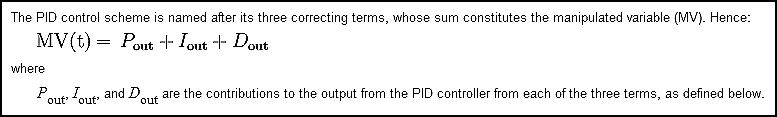where u(t) is the output of the controller.

The mathematical formula given above can be applied to just about any PID controller. These devices are used extensively in almost any household appliance or in any manufacturing process in the industry. According to the specific type of the process, different coefficient values are used for P, I and D control respectively. Hence, an enormous variety of output functions with the PID controller can be achieved.

Here the analogy between the principle of operation of the PID controller and the astrological chart of a person becomes more obvious. The action of the proportional controller is corresponding to that of the Sun, the integral controller is analogous to the Moon and the action of the differential controller is similar to the Ascendant. The error between the SP and the PV is like the deviation of the man’s behaviour from his preordained path for spiritual development. The coefficients for P, I and D control are directly proportional to the astrological signs, in which the respective planets are situated. A certain mathematical weight can be given to each sign of the Zodiac, depending on its specific strength in the chart. The weight of the coefficients also can be calculated mathematically, according to the affinities between the type of the coefficient and the astrological sign in which it is located, any other planets located in that sign, as well as any aspects that it receives. Therefore, if we substitute the specific coefficients, characteristic for a particular person in the equation above, we can obtain a simplified mathematical formula describing his behaviour.

Of course, we have to be aware that our method and the obtained formula are extremely simplified. We take into account only the three major factors in any astrological chart – the Ascendant, the Sun and the Moon. However, this approach is a step in the right direction. Based on these three major points in the chart, every experienced astrologer would be able to delineate about 30% of his subject’s personality. If in addition to that we include the influences of the other planets by signs, house positions and aspects in the formula, we would be able to obtain a model which is about 90% accurate. The importance of this conclusion lies in the fact that it shows the way in which to create a very real and credible mathematical (and therefore scientific) model, describing the human behaviour.

Nowadays, millions of PID controllers are already designed, manufactured and commissioned worldwide. Using the same concept, the behaviour of people can also be modelled scientifically employing astrology studies. Subsequently, computer programs can be developed that predict the human behaviour, based on this mathematical model. The only factor that cannot be taken into consideration with this concept is the action caused by the human free will. This is something that cannot be predicted mathematically with the technology that we have today. However, bearing in mind that for an the overwhelming majority of people, the free will component does not account for more than 5-10% of their choices, this model still can produce very reliable and accurate results. Such a program can predict people’s behaviour with an accuracy that is not worse than for example the accuracy of the weather forecast today.

It is interesting, that most people these days believe that weather predictions are scientific, but a lot of them are not sure if astrology is a science too. More often than not, the ones that are most sceptical are the ones that know very little about it. Based on the above mentioned model, human behaviour can be predicted with the same degree of accuracy if not better, as the weather predictions are done today. We should mention here in parenthesis that the weather predictions are only accurate for short periods of time ahead. If we try to devise what the weather will be like in a few months or a few years in advance, scientists can only make educated guesses. In striking contrast, many astrologers have been much more accurate in their predictions for far longer periods of time ahead. But this scepticism will not last. In time everything will be put in their correct places. In the future, astrology together with mathematics undoubtedly will be regarded as the core of all sciences. I am hopeful that this article will make its small contribution to assist this process.